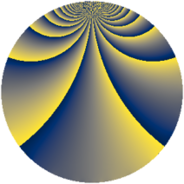# Properties

 Label 1078.2.oLevel $1078$ Weight $2$ Character orbit 1078.o Rep. character $\chi_{1078}(153,\cdot)$ Character field $\Q(\zeta_{14})$ Dimension $336$ Sturm bound $336$

# Related objects

## Defining parameters

 Level: $$N$$ $$=$$ $$1078 = 2 \cdot 7^{2} \cdot 11$$ Weight: $$k$$ $$=$$ $$2$$ Character orbit: $$[\chi]$$ $$=$$ 1078.o (of order $$14$$ and degree $$6$$) Character conductor: $$\operatorname{cond}(\chi)$$ $$=$$ $$539$$ Character field: $$\Q(\zeta_{14})$$ Sturm bound: $$336$$

## Dimensions

The following table gives the dimensions of various subspaces of $$M_{2}(1078, [\chi])$$.

Total New Old
Modular forms 1032 336 696
Cusp forms 984 336 648
Eisenstein series 48 0 48

## Trace form

 $$336 q + 56 q^{4} + 56 q^{9} + O(q^{10})$$ $$336 q + 56 q^{4} + 56 q^{9} + 6 q^{11} - 8 q^{14} - 20 q^{15} - 56 q^{16} + 28 q^{20} + 14 q^{22} + 52 q^{23} + 56 q^{25} + 28 q^{26} + 84 q^{27} - 56 q^{36} + 16 q^{37} + 28 q^{38} - 116 q^{42} - 6 q^{44} + 112 q^{45} - 56 q^{47} + 72 q^{49} - 112 q^{53} - 168 q^{55} - 20 q^{56} - 60 q^{58} - 28 q^{59} + 20 q^{60} + 56 q^{64} + 8 q^{67} - 56 q^{69} + 4 q^{70} - 8 q^{71} - 46 q^{77} - 32 q^{78} - 160 q^{81} + 60 q^{86} + 14 q^{88} - 168 q^{89} + 164 q^{91} + 32 q^{92} + 24 q^{93} - 144 q^{99} + O(q^{100})$$

## Decomposition of $$S_{2}^{\mathrm{new}}(1078, [\chi])$$ into newform subspaces

The newforms in this space have not yet been added to the LMFDB.

## Decomposition of $$S_{2}^{\mathrm{old}}(1078, [\chi])$$ into lower level spaces

$$S_{2}^{\mathrm{old}}(1078, [\chi]) \cong$$ $$S_{2}^{\mathrm{new}}(539, [\chi])$$$$^{\oplus 2}$$